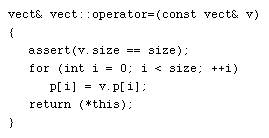Write a member function that overloads the assignment operator for the matrix class so that the operator assigns one two-dimensional array to another. Before looking at overloading the assignment operator, you may want to review the use of reference declaration.
Look at the binary operator overloading by focusing on the safe array class from the Building Classes in C++ course. It would be convenient to assign one array to another. We can specify the behavior of assignment by overloading the assignment operator (`=`). It is good style to maintain consistency with standard usage and avoid creating personal algebras.

## Safe Array in C++

Here is the implementation of a safe array that was used in Building Classes in C++:
```//Implementation of a safe array type vect
class vect {
public:
explicit vect(int n = 10);
~vect() { delete []p; }
int& element(int i);        //access p[i]
int  ub() const
{ return (size - 1); }  //upper bound
private:
int*  p;
int   size;
};

vect::vect(int n) : size(n){
assert(n > 0);
p = new int[size];
assert(p);
}

int& vect::element(int i){
assert (i >= 0 && i < size);
return p[i];
}
```

Following is the code to create a safearray in C++.
```#include<oaidl.h>

double data; // some sample data to write into the created safearray
SAFEARRAYBOUND  Bound;
Bound.lLbound   = 0;
Bound.cElements = 10;

double HUGEP *pdFreq;
HRESULT hr = SafeArrayAccessData(*saData, (void HUGEP* FAR*)&pdFreq);
if (SUCCEEDED(hr)) {
// copy sample values from data[] to this safearray
for (DWORD i = 0; i < 10; i++){
*pdFreq++ = data[i];
}
}
}
```

When you are finished free the pointer using the following code.
```  SAFEARRAY* saData;
CreateSafeArray(&saData); // Create the safe array
// use the safearray
...
...

// Call the SafeArrayDestroy to destroy the safearray
saData = NULL; // set the pointer to NULL
```

New Assignment
We can add the following member function, which overloads the assignment operator for the `vect class`:

Any time a `class` uses `new` to construct objects, it should provide an explicitly overloaded assignment operator. This advice is analogous to our rule that such a `class` provide an explicit copy constructor.
The compiler-provided default assignment operator semantics would, in most cases, give spurious behavior. This leads to a suggested normal form for classes with heap-managed memory. This normal form rule applies as well to reference counted classes. The reason the assignment operator returns a reference is to allow assignment to work efficiently. This requires lvalue semantics.

In C++ you can give special meanings to operators, when they are used with user-defined classes. This is called operator overloading. You can implement C++ operator overloads by providing special member-functions on your classes that follow a particular naming convention. For example, to overload the + operator for your class, you would provide a member-function named operator+ on your class. The following set of operators is commonly overloaded for user-defined classes:

1. = (assignment operator)
2. + - * (binary arithmetic operators)
3. += -= *= (compound assignment operators)
4. == != (comparison operators)

### Program dissection

Move your mouse cursor over the 1) operator= 2) assert and 3) for loop to to read a description of their purpose.The "operator=()" function returns reference to "vect" and has one explicit argument of type reference to "vect". The first argument of the assignment operator is the implicit argument. The function could have been written to return void, but then it would not have allowed multiple assignment. This guarantees that the sizes are compatible. The explicit argument "v.p[]" will be the right side of the assignment. The implicit argument "p[]" will be the left side of the assignment. The self-referential pointer is dereferenced and passed back as the value of the expression. This allows multiple assignment with right-to-left associativity to be defined. operator=()

and

### Program Dissection

```vect& vect::operator=(const vect& v)
{
assert(v.size == size);
for (int i = 0; i < size; ++i)
p[i] = v.p[i];
return (*this);
}
```

```vect& vect::operator=(const vect& v)
```

The `operator=()` function returns reference to `vect` and has one explicit argument of type reference to `vect`. The first argument of the assignment operator is the implicit argument. The function could have been written to return `void`, but then it would not have allowed multiple assignment.

```assert(v.size == size);
```

This guarantees that the sizes are compatible.
```for (int i = 0; i < size; ++i)
p[i] = v.p[i];
return (*this);
```

The explicit argument `v.p[]` will be the right side of the assignment; the implicit argument `p[]` will be the left side of the assignment. The self-referential pointer is dereferenced and passed back as the value of the expression. This allows multiple assignment with right-to-left associativity to be defined.
Now with the `class vect`, the following expressions are meaningful:
```a = b;                  //a, b are type vect
a = b= c;             //a, b, c are type vect
a = vect(data, DSIZE);  //convert array data[DSIZE]
```